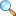# MTH211: Discrete Mathematics (Fall 2020)

Discrete Mathematics is branch of Mathematics which deals with discrete structures like logic. sequences, graphs, relations in contrast to Calculus. where we enjoy the continuity of functions and the set of real numbers. This course is introduction to discrete structures which are not the part of main stream courses. Discrete Mathematics has applications in Computer Science. Economics and Decision Making etc. This course will help the student to understand the basic logical system, counting principles, relations. solution of recurrence relation. solution of the problems which can be translated in simple diagrams namely Graphs and Trees and the comparison between two algorithms in the sense of efficiency. The objective of the course is to inculcate in student the skills required for decision making in non- continuous situations.

Logical forms and equivalences, conditional statements, validity of arguments, quantiﬁers, multiplication rule, inclusion-exclusion formulae, permutations and combinations, pigeonhole principle, binary relations. n-ary relations, equivalence relations, partial order relations, recursive relation, solution of recurrence relation by iteration and by root method, graphs .matrix representations of graphs, graphs isomorphism, trees, connectivity, Eulerian and Hamiltonian paths, spanning trees and shortest path problem. revisiting the graphs of power function, ﬂoor function, increasing and decreasing functions, big 0, little 0 and w notations, orders of polynomial functions, orders of simple algorithms, efﬁciency of an algorithm, exponential and logarithmic orders, efﬁciency of binary search algorithm.

Here is the list of contents covered in different lecture with reference to book.

Lectures

• Lecture 1 to 6 are based upon “Chapter 4: Logic and Propositional Calculus” of .
• Lecture 7 to 10 are based upon “Chapter 3: Functions and Algorithms” of .

#### Quiz/Assignment

• Quiz/Assignment 04 | Download PDF |View OnlinePlease click on View Online to see inside the PDF.

1. M.L. Lipson, S. Lipschutz, Schaum’s Outline of Theory and Problems of Discrete Mathematics, 3rd Edition, McGraw-Hill, New York, 1997.
2. K.H. Rosen, Discrete Mathematics and its Application, 6th Edition, McGraw-Hill, New York, 2007.
3. K.R. Parthasarathy, Basic Graph Theory. McGraw-Hill. 1994.
4. B. Bollobas, Graph Them-y. Springer Verlag. New York, 1979.
5. B. Kolman, R.C. Busby, S.C. Ross, Discrete Mathematical Structure, Prentice-Hall of India, New Delhi, 5th Edition. 2008.
6. A. Tucker, Applied Combinatorics, John Wiley and Sons. Inc New York, 2002.
7. R. Diestel, Graph Theory, 4th edition, Springer-Verlag. New York. 2010.
8. N.L. Brigs, Discrete Mathematics, Oxford University Press. 2003
9. K.A. Ross, C.R.B. Wright, Discrete Mathematics, Prentice Hall. New Jersey, 2003.
• atiq/fa20-mth211